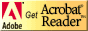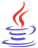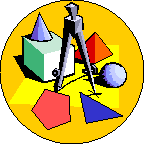# Standardized Test Preparation and Tips for Success

## Strand Resources: Geometry and Spatial SenseCT4ME has identified resources related to each benchmark of the Ohio Mathematics Academic Content Standards that students should have mastered by the end of the grades 8-10 program.

Identify the benchmark (A-I) for review in Geometry and Spatial Sense.  Below the benchmark, you will find Web resources for reviewing the concept and practice problems.

Students should be able to review on their own using these resources.  If you are not an Ohio educator, these resources can still benefit your test prep efforts, as benchmarks in other states are similarly stated.

Geometry and Spatial Sense (pdf)  This test prep booklet (about 200 KB) is designed to help you monitor your progress toward mastery of each benchmark in this strand.  You can print the entire booklet, or just those pages for benchmarks you need to work on.  Write notes in the booklet next to each resource you use in your review.If needed, download Adobe Acrobat Reader, free software for viewing and printing PDF files.You might need to download Java, a free plug-in to interact with virtual manipulatives on this page.Other strand resources:

Number, Number Sense, and Operations ]   [ Measurement ]   [ Geometry and Spatial Sense ]   [ Patterns, Functions, and Algebra ]   [ Data Analysis and Probability ]   [ Mathematical Processes ]### What is Geometry?### Benchmarks for Geometry and Spatial Sense

Benchmarks in this strand have been correlated to the Common Core State Standards (CCSS) for Mathematics for the Conceptual Category (CC), Domain (D), and Cluster (C).NOTE: As general resources for all Geometry and Spatial Sense benchmarks, students might consult the following:

• Math Open Reference includes a free geometry textbook for high school learners by John Page.  There are topics in plane geometry, coordinate geometry, and solid geometry (e.g., cylinders, cubes). Its focus is on using interactive materials and animations to develop concepts.  Students can manipulate numerous interactive applets with at least one accompanying nearly every concept that is presented.

A.  Formally define geometric figures.

CCSS: CC: Geometry, D: Congruence, C: Experiment with transformations in the plane.

CCSS: CC: Geometry, D: Circles, C: Understand and apply theorems about circles, and C: Find arc lengths and areas of sectors of circles.

Math.com: Geometry basics.  Figures are defined with lessons, examples, workout quizzes, and unit quizzes

Mathwords.com: interactive math dictionary with words, terms, formulas, pictures, diagrams, tables, and examples

ExploreLearning Gizmos (Virtual Manipulatives): Quadrilaterals and other Polygons.

Geometry 3-D Shapes is an interactive from Annenberg Learner intended for grades 6-8, although other grades can benefit.  Students can interactively learn about three-dimensional shapes, calculate surface area and volume, and discover some of the mathematical properties of shapes. It comes with a glossary, tutorials, and an interactive test.

B.  Describe and apply the properties of similar and congruent figures; and justify conjectures involving similarity and congruence.

CCSS: CC: Geometry, D: Similarity, Right Triangles, and Trigonometry, C: Understand similarity in terms of similarity transformations, and C: Prove theorems involving similarity.

CCSS: grade 8, D: Number System, C: Understand congruence and similarity using physical models, transparencies, or geometry software.

AlgebraLab.org:  Lesson and fill in the blank practice problems, including word problems.  Be sure to show the related AlgebraLab documents for additional practice problems on each topic.

Math.com: Congruent and similar figures including lesson and workout problems.

Utah State University, National Library of Virtual Manipulatives: investigate methods for determining congruent triangles.  Explore SSS, SAS, ASA, SSA.  Note SSA does not guarantee congruent triangles.

C.  Recognize and apply angle relationships in situations involving intersecting lines, perpendicular lines and parallel lines.

CCSS: CC: Geometry, D: Congruence, C: Prove geometric theorems.

CCSS: grade 8, D: Number System, C: Understand congruence and similarity using physical models, transparencies, or geometry software.

AlgebraLab.org:  Lesson and fill in the blank practice problems.  Be sure to show the related AlgebraLab documents for additional practice problems on each topic

Math.com: Angles and Intersecting Lines including lesson and workout problems

Shodor Interactivate: Angles formed by parallel lines and one or two transversals.  This activity allows users to practice angle vocabulary.

Walter Fendt's Sum of Angles in a Triangle, Virtual ManipulativePlay the YouTube video from the Ohio Resource Center Tutorials for High School Mathematics:

D.  Use coordinate geometry to represent and examine the properties of geometric figures.

CCSS: CC: Geometry, D: Expressing Geometric Properties with Equations, C: Use coordinates to prove simple geometric theorems algebraically.

Math Warehouse: Using the coordinate plane in proofs is an interactive demonstrating how to do coordinate proofs.  Do the proofs (perpendicular segments, trapezoid is not isosceles, and rectangle) and see the answers.

E.  Draw and construct representations of two- and three-dimensional geometric objects using a variety of tools, such as straightedge, compass and technology.

CCSS: CC: Geometry, D: Congruence, C: Make geometric constructions.

CCSS: CC: grade 7, D: Geometry, C: Draw, construct, and describe geometrical figures and describe the relationships between them.

Mathematics Open Reference: Visual demonstrations of how to do various Euclidean constructions with compass and straightedge.  (highly recommended)

F.  Represent and model transformations in a coordinate plane and describe the results.

CCSS: CC: Geometry, D: Congruence, C: Experiment with transformations in the plane.

CCSS: grade 8, D: Number System, C: Understand congruence and similarity using physical models, transparencies, or geometry software.

Utah State University, National Library of Virtual Manipulatives: show axes, create objects with pattern block pieces, then apply and explore the following:

G.  Prove or disprove conjectures and solve problems involving two- and three-dimensional objects represented within a coordinate system.

CCSS: CC: Geometry, D: Congruence, C: Prove geometric theorems.

CCSS: CC: Geometry, D: Expressing Geometric Properties with Equations, C: Use coordinates to prove simple geometric theorems algebraically.

Utah State University, National Library of Virtual Manipulatives: Platonic Solids, Verify Vertices, Faces, Edges of five Solids

H.  Establish the validity of conjectures about geometric objects, their properties and relationships by counter-example, inductive and deductive reasoning, and critiquing arguments made by others.

CCSS: CC: Geometry, D: Congruence, C: Understand congruence in terms of rigid motions, and C: Prove geometric theorems.

CCSS: CC: Geometry, D: Similarity, Right Triangles, and Trigonometry, C: Prove theorems involving similarity.

CCSS: CC: Geometry, D: Expressing Geometric Properties with Equations, C: Use coordinates to prove simple geometric theorems algebraically.

CCSS: grade 8, D: Number System, C: Understand and apply the Pythagorean Theorem.

Utah State University, National Library of Virtual Manipulatives: walk through a visual proof of the Pythagorean theorem.

I.  Use right triangle trigonometric relationships to determine lengths and angle measures.

CCSS: CC: Geometry, D: Similarity, Right Triangles, and Trigonometry, C: Define trigonometric ratios and solve problems involving right triangles.

Are you wondering why a2 + b2 = c2 in the right triangle?See the GeoGebra Book of Proofs Without Words for the Pythagorean Theorem by Steve Phelps, which is a series of manipulatives.Play videos 9-13 on Pythagorean Theorem, sine, cosine, and tangent ratios at GCSE Maths Tutor: Introducing Trigonometry:

• #9 - Pythagoras'Theorem - Part 1:  "This video looks at the Pythagoras' Theorem. An appreciation of the theorem is given graphically and algebraically. Following is a formal proof, explained in some detail. Before problems are attempted, a little time is spent on 'rounding to decimal places'. The video ends with three worked examples on the Pythagoras Theorem."
• #10 - Pythagoras'Theorem - Part 2: "This video is the second of two about Pythagoras' Theorem. Another proof is described. This one uses similar triangles.
The concept of 'triples' (right-angled triangles with integral number sides) is explored.
• #11 - The Sine Ratio: "The Sine Ratio is defined and its limits explored. The sine values of common angles 30, 45 and 60 degrees are described The tutorial includes worked examples of three common problem types."
• #12 - The Cosine Ratio: "The cosine is defined and its limits explored. The values of common angles 30, 45 and 60 degrees are described.  The tutorial includes worked examples of three common problem types."
• #13 - The Tangent Ratio: "The tangent ratio is defined and its limits explored. The values of common angles 30, 45 and 60 degrees are described.  The tutorial includes worked examples of three common problem types."Play the YouTube video from the Ohio Resource Center Tutorials for High School Mathematics:

AlgebraLab.org:  Lesson and fill in the blank practice problems.

Mathguide.com: Pythagorean theorem quizmaster.  The following are fill in and check answers, which are rounded to the nearest tenth.  The formula is presented with the following:

Math.com: Right Triangle and Pythagorean Theorem including lesson and workout problemsAre you ready for the test?

#### References

*Ohio Mathematics Academic Content Standards (2001). http://www.ode.state.oh.us/GD/Templates/Pages/ODE/ODEPrimary.aspx?page=2&TopicID=1696&TopicRelationID=1704 [URL is no longer active.]

Ohio Mathematics Academic Content Standards Crosswalk, K-8 and High School (2010).  https://web.archive.org/web/20180206120934/http://education.ohio.gov/getattachment/Topics/Ohio-s-New-Learning-Standards/Mathematics/Transition-Tools-Ohio-s-New-Learning-Standards-K-1/Alignment-Toolkit-Phase-1-Gap-Analysis/HS-crosswalk-mathematics-4-17-12.pdf.aspx  [Revised 4/17/2012].

*Note 2019: The current academic content standards for mathematics are now available at http://education.ohio.gov/Topics/Learning-in-Ohio/Mathematics/Ohio-s-Learning-Standards-in-MathematicsSee other strand resources:

Number, Number Sense, and Operations ]   [ Measurement ]   [ Geometry and Spatial Sense ]   [ Patterns, Functions, and Algebra ]   [ Data Analysis and Probability ]   [ Mathematical Processes ]See related pages: [ Standardized Test Preparation and Tips for Success (first  page)]  [ OGT Introduction ]